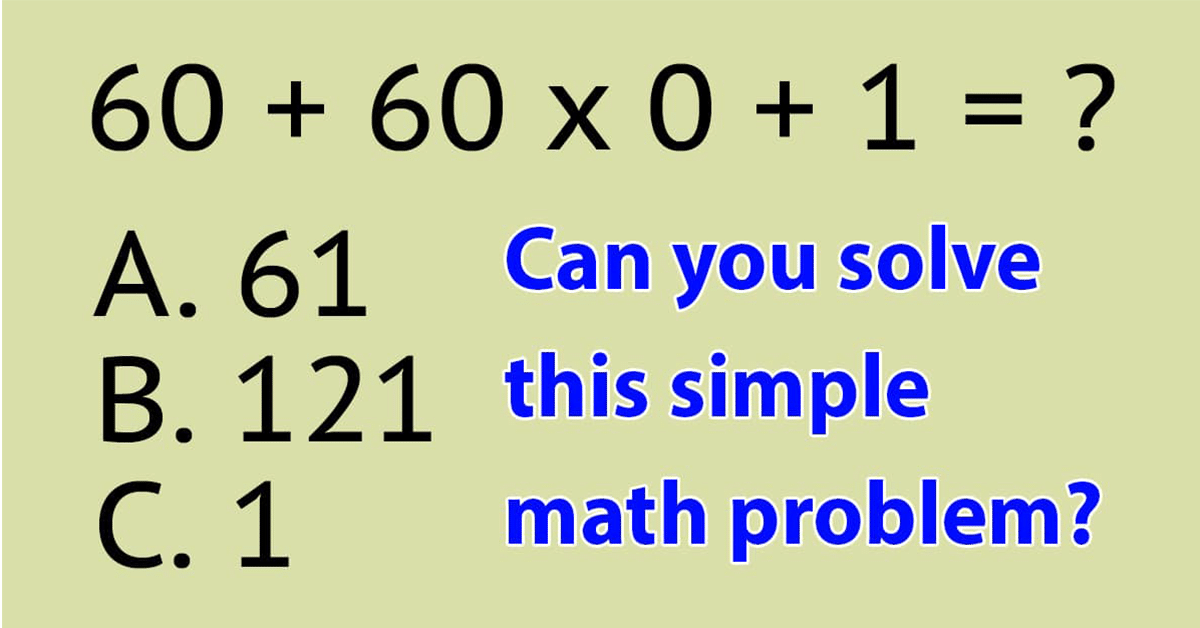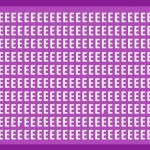in

# Let’s find out if you’re smart enough to solve this tricky math problemCan you find the correct number?

At the top of the picture we see the task and then four possible answers.

Which solution do you think is the correct one?

How did you come up with it?

It is important to try to remember the correct order to solve a math problem to get the right answer.

Below you can see the correct answer!

The correct answer is A: 61.

Why is that?

Well, first of all, we have to start by solving the multiplication, 60 x 0, which will be 0.

So this leaves us with 60 + 0 + 1 and so, it becomes quite easy to see that the correct answer is 61.

But if you forget you must always start with the multiplication, then it gets tricky!

Did you solve the problem correctly? Congratulations to you!

Now click that SHARE button and see how many of your friends can solve it as well!### Letters “F” Are Hidden Among The Es